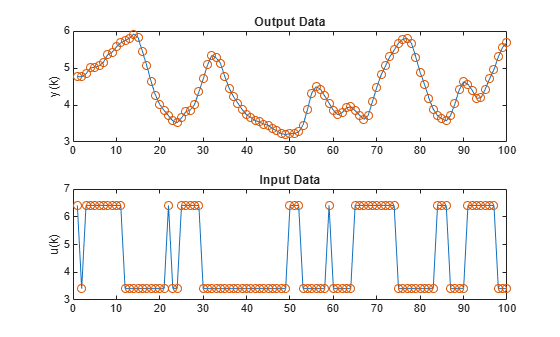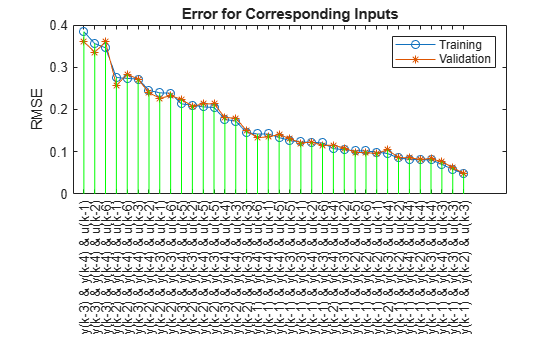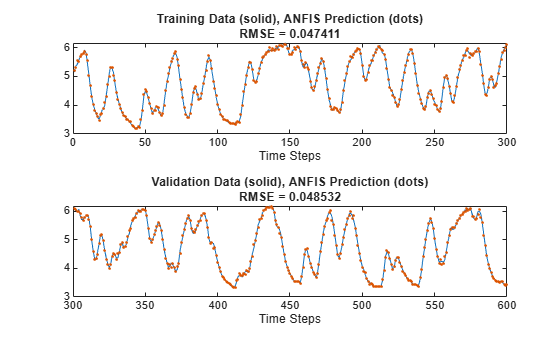Nonlinear System Identification

This example shows how to perform dynamic system identification by using a linear ARX and a nonlinear ANFIS model.

The data set used in this example for ANFIS and ARX modeling is from a "Feedback's Process Trainer PT 326" laboratory device . The device functions like a hair dryer: air is fanned through a tube and heated at the inlet. A thermocouple measures the air temperature. The input $\mathit{u}\left(\mathit{k}\right)$ is the voltage over a mesh of resistor wires to heat incoming air and the output $\mathit{y}\left(\mathit{k}\right)$ is the outlet air temperature.Load the test data and plot the input and output.

data_n = length(y);
output = y;
input = [[0; y(1:data_n-1)] ...
[0; 0; y(1:data_n-2)] ...
[0; 0; 0; y(1:data_n-3)] ...
[0; 0; 0; 0; y(1:data_n-4)] ...
[0; u(1:data_n-1)] ...
[0; 0; u(1:data_n-2)] ...
[0; 0; 0; u(1:data_n-3)] ...
[0; 0; 0; 0; u(1:data_n-4)] ...
[0; 0; 0; 0; 0; u(1:data_n-5)] ...
[0; 0; 0; 0; 0; 0; u(1:data_n-6)]];
data = [input output];
data(1:6,:) = [];
input_name = ["y(k-1)","y(k-2)","y(k-3)","y(k-4)",...
"u(k-1)","u(k-2)","u(k-3)","u(k-4)","u(k-5)","u(k-6)"];
index = 1:100;

figure
subplot(2,1,1)
plot(index,y(index),'-',index,y(index),'o')
title('Output Data')
ylabel('y(k)')
subplot(2,1,2)
plot(index,u(index),'-',index,u(index),'o')
title('Input Data')
ylabel('u(k)')The data points reflect a sample time of 0.08 seconds. The input $\mathit{u}\left(\mathit{k}\right)$ is a binary random signal shifting between 3.41 and 6.41. The probability of shifting the input at each sample is 0.2. The data set contains 1000 input/output data points. These plots show the output temperature $\mathit{y}\left(\mathit{k}\right)$ and input voltage $\mathit{u}\left(\mathit{k}\right)$ for the first 100 time steps.

Identify ARX Model

An ARX model is a linear model of the following form:

$y\left(k\right)+{\mathit{a}}_{1}\cdot \mathit{y}\left(k-1\right)+...+{\mathit{a}}_{\mathit{m}}\cdot y\left(k-m\right)={\mathit{b}}_{1}\cdot \mathit{u}\left(k-d\right)+...+{\mathit{b}}_{\mathit{n}}\cdot \mathit{u}\left(k-d-n+1\right)$

Here:

• $\mathit{y}\left(\mathit{k}\right)$ and $\mathit{u}\left(\mathit{k}\right)$ are mean-subtracted versions of the original data.

• ${\mathit{a}}_{\mathit{i}}$ and ${\mathit{b}}_{\mathit{j}}\text{\hspace{0.17em}}$ are linear parameters.

• $\mathit{m}$, $\mathit{n}$, and $\mathit{d}$ are three integers that exactly specify the ARX model.

To find an ARX model for the dryer device, first divide the data set into a training ($\mathit{k}$ = 1 to 300) and a validation ($\mathit{k}$ = 301 to 600) set.

trn_data_n = 300;
total_data_n = 600;
z = [y u];
z = dtrend(z);
ave = mean(y);
ze = z(1:trn_data_n,:);
zv = z(trn_data_n+1:total_data_n,:);
T = 0.08;

Perform an exhaustive search to find the best combination of $\mathit{m}$, $\mathit{n}$, and $\mathit{d}$, allowing each integer to change from 1 to 10 independently. To perform the search and select the ARX parameters, use the arxstruc (System Identification Toolbox) and selstruc (System Identification Toolbox) functions.

% Run through all different models.
V = arxstruc(ze,zv,struc(1:10,1:10,1:10));
% Find the best model.
nn = selstruc(V,0);
% Display model parameters
disp(['[m n d] = ' num2str(nn)])
[m n d] = 5  10   2

The best ARX model has $\mathit{m}$ = 5, $\mathit{n}$ = 10, and $\mathit{d}$ = 2. Create with a training root mean squared error (RMSE) of 0.1122 and a validation RMSE of 0.0749. Plot the original $\mathit{y}\left(\mathit{k}\right)$ along with this ARX model.

Create and simulate an ARX model with these parameters.

th = arx(ze,nn);
th.Ts = 0.08;
u = z(:,2);
y = z(:,1) + ave;
yp = sim(u,th) + ave;

Plot the ARX model output against the training and validation data. The training root mean squared error (RMSE) is 0.1121 and the validation RMSE is 0.0748.

figure
subplot(2,1,1)
index = 1:trn_data_n;
plot(index,y(index),index,yp(index), '.')
rmse = norm(y(index)-yp(index))/sqrt(length(index));
title("Training Data (solid), ARX Prediction (dots)" ...
+ newline + "RMSE = " + num2str(rmse))
xlabel('Time Steps')

subplot(2,1,2)
index = (trn_data_n+1):(total_data_n);
plot(index,y(index),index,yp(index),'.')
rmse = norm(y(index)-yp(index))/sqrt(length(index));
title("Validation Data (solid), ARX Prediction (dots)" ...
+ newline + "RMSE = " + num2str(rmse))
xlabel('Time Steps')Identify ANFIS Model

The ARX model is linear and can perform model structure and parameter identification rapidly. The performance in the previous plots appears to be satisfactory. However, if you want better performance, you can try a nonlinear model such as an adaptive neuro-fuzzy inference system (ANFIS).

To use an ANFIS for system identification, first determine which variables to use for the input arguments. For simplicity, use 10 input candidates ($\mathit{y}\left(\mathit{k}-1\right)$, $\mathit{y}\left(\mathit{k}-2\right)$, $\mathit{y}\left(\mathit{k}-3\right)$, $\mathit{y}\left(\mathit{k}-4\right)$, $\mathit{u}\left(\mathit{k}-1\right)$, $\mathit{u}\left(\mathit{k}-2\right)$, $\mathit{u}\left(\mathit{k}-3\right)$, $\mathit{u}\left(\mathit{k}-4\right)$, $\mathit{u}\left(\mathit{k}-5\right)$, and $\mathit{u}\left(\mathit{k}-6\right)$). Use $\mathit{y}\left(\mathit{k}\right)$ as the output.

Perform a sequential forward search of the inputs using the function sequentialSearch. This function selects each input variable sequentially to optimize the RMSE.

trn_data_n = 300;
trn_data = data(1:trn_data_n,:);
val_data = data(trn_data_n+1:trn_data_n+300,:);
[~,elapsed_time] = sequentialSearch(3,trn_data,val_data,input_name);
Selecting input 1 ...
ANFIS model 1: y(k-1) --> trn=0.2043, val=0.1888
ANFIS model 2: y(k-2) --> trn=0.3819, val=0.3541
ANFIS model 3: y(k-3) --> trn=0.5245, val=0.4903
ANFIS model 4: y(k-4) --> trn=0.6308, val=0.5977
ANFIS model 5: u(k-1) --> trn=0.8271, val=0.8434
ANFIS model 6: u(k-2) --> trn=0.7976, val=0.8087
ANFIS model 7: u(k-3) --> trn=0.7266, val=0.7349
ANFIS model 8: u(k-4) --> trn=0.6215, val=0.6346
ANFIS model 9: u(k-5) --> trn=0.5419, val=0.5650
ANFIS model 10: u(k-6) --> trn=0.5304, val=0.5601
Currently selected inputs: y(k-1)

Selecting input 2 ...
ANFIS model 11: y(k-1) y(k-2) --> trn=0.1085, val=0.1024
ANFIS model 12: y(k-1) y(k-3) --> trn=0.1339, val=0.1283
ANFIS model 13: y(k-1) y(k-4) --> trn=0.1542, val=0.1461
ANFIS model 14: y(k-1) u(k-1) --> trn=0.1892, val=0.1734
ANFIS model 15: y(k-1) u(k-2) --> trn=0.1663, val=0.1574
ANFIS model 16: y(k-1) u(k-3) --> trn=0.1082, val=0.1077
ANFIS model 17: y(k-1) u(k-4) --> trn=0.0925, val=0.0948
ANFIS model 18: y(k-1) u(k-5) --> trn=0.1533, val=0.1531
ANFIS model 19: y(k-1) u(k-6) --> trn=0.1952, val=0.1853
Currently selected inputs: y(k-1) u(k-4)

Selecting input 3 ...
ANFIS model 20: y(k-1) u(k-4) y(k-2) --> trn=0.0808, val=0.0822
ANFIS model 21: y(k-1) u(k-4) y(k-3) --> trn=0.0806, val=0.0836
ANFIS model 22: y(k-1) u(k-4) y(k-4) --> trn=0.0817, val=0.0855
ANFIS model 23: y(k-1) u(k-4) u(k-1) --> trn=0.0886, val=0.0912
ANFIS model 24: y(k-1) u(k-4) u(k-2) --> trn=0.0835, val=0.0843
ANFIS model 25: y(k-1) u(k-4) u(k-3) --> trn=0.0609, val=0.0604
ANFIS model 26: y(k-1) u(k-4) u(k-5) --> trn=0.0848, val=0.0867
ANFIS model 27: y(k-1) u(k-4) u(k-6) --> trn=0.0890, val=0.0894
Currently selected inputs: y(k-1) u(k-3) u(k-4)This plot shows all combinations of inputs tried by sequentialSearch. The search selects $\mathit{y}\left(\mathit{k}-1\right)$, $\mathit{u}\left(\mathit{k}-3\right)$, and $\mathit{u}\left(\mathit{k}-4\right)$ as inputs, as the model with these inputs has the lowest training RMSE (0.609) and validation RMSE (0.604).

Alternatively, use an exhaustive search on all possible combinations of the input candidates. As before, search for three inputs out of the 10 candidates. You can use the function exhaustiveSearch for such a search; however, this function tries all possible combinations of candidates, $\left(\genfrac{}{}{0}{}{10}{3}\right)=120$ in this case.

Instead of exhaustiveSearch, use custom code to search through a subset of these combinations. For this example, do not select any input combination exclusively from the inputs or exclusively from the outputs.

As a reasonable guess, select input combinations with two output values and one input value, which produces 36 possible input combinations. Define groups for selecting input indices: two groups for selecting an output and one group for selecting an input.

group1 = [1 2 3 4];	% y(k-1), y(k-2), y(k-3), y(k-4)
group2 = [1 2 3 4];	% y(k-1), y(k-2), y(k-3), y(k-4)
group3 = [5 6 7 8 9 10];	% u(k-1) through u(k-6)

Specify parameters and options for training.

anfis_n = 6*length(group3);
index = zeros(anfis_n,3);
trn_error = zeros(anfis_n,1);
val_error = zeros(anfis_n,1);

% Create option set for generating initial FIS.
genOpt = genfisOptions('GridPartition','NumMembershipFunctions',2, ...
'InputMembershipFunctionType','gbellmf');
% Create option set for anfis function and set options that remain
% constant for different training scenarios.
anfisOpt = anfisOptions('EpochNumber',1,...
'InitialStepSize',0.1,...
'StepSizeDecreaseRate',0.5,...
'StepSizeIncreaseRate',1.5,...
'DisplayANFISInformation',0,...
'DisplayErrorValues',0,...
'DisplayStepSize',0,...
'DisplayFinalResults',0);

Train ANFIS model for each input combination.

model = 1;
for i = 1:length(group1)
for j = i+1:length(group2)
for k = 1:length(group3)
% Create input combinations.
in1 = input_name(group1(i));
in2 = input_name(group2(j));
in3 = input_name(group3(k));
index(model, :) = [group1(i) group2(j) group3(k)];
trn_data = data(1:trn_data_n, ...
[group1(i) group2(j) group3(k) size(data,2)]);
val_data = data(trn_data_n+1:trn_data_n+300, ...
[group1(i) group2(j) group3(k) size(data,2)]);

% Create the initial FIS structure.
in_fis = genfis(trn_data(:,1:end-1),trn_data(:,end),genOpt);

% Set the initial FIS and validation data for ANFIS training.
anfisOpt.InitialFIS = in_fis;
anfisOpt.ValidationData = val_data;

% Train the ANFIS system.
[~,t_err,~,~,c_err] = anfis(trn_data,anfisOpt);
trn_error(model) = min(t_err);
val_error(model) = min(c_err);
fprintf('ANFIS model = %d: %s %s %s',model,in1,in2,in3);
fprintf(' --> trn=%.4f,',trn_error(model));
fprintf(' val=%.4f',val_error(model));
fprintf('\n');
model = model+1;
end
end
end
ANFIS model = 1: y(k-1) y(k-2) u(k-1)
--> trn=0.0990,
val=0.0962
ANFIS model = 2: y(k-1) y(k-2) u(k-2)
--> trn=0.0852,
val=0.0862
ANFIS model = 3: y(k-1) y(k-2) u(k-3)
--> trn=0.0474,
val=0.0485
ANFIS model = 4: y(k-1) y(k-2) u(k-4)
--> trn=0.0808,
val=0.0822
ANFIS model = 5: y(k-1) y(k-2) u(k-5)
--> trn=0.1023,
val=0.0991
ANFIS model = 6: y(k-1) y(k-2) u(k-6)
--> trn=0.1021,
val=0.0974
ANFIS model = 7: y(k-1) y(k-3) u(k-1)
--> trn=0.1231,
val=0.1206
ANFIS model = 8: y(k-1) y(k-3) u(k-2)
--> trn=0.1047,
val=0.1085
ANFIS model = 9: y(k-1) y(k-3) u(k-3)
--> trn=0.0587,
val=0.0626
ANFIS model = 10: y(k-1) y(k-3) u(k-4)
--> trn=0.0806,
val=0.0836
ANFIS model = 11: y(k-1) y(k-3) u(k-5)
--> trn=0.1261,
val=0.1311
ANFIS model = 12: y(k-1) y(k-3) u(k-6)
--> trn=0.1210,
val=0.1151
ANFIS model = 13: y(k-1) y(k-4) u(k-1)
--> trn=0.1420,
val=0.1353
ANFIS model = 14: y(k-1) y(k-4) u(k-2)
--> trn=0.1224,
val=0.1229
ANFIS model = 15: y(k-1) y(k-4) u(k-3)
--> trn=0.0700,
val=0.0765
ANFIS model = 16: y(k-1) y(k-4) u(k-4)
--> trn=0.0817,
val=0.0855
ANFIS model = 17: y(k-1) y(k-4) u(k-5)
--> trn=0.1337,
val=0.1405
ANFIS model = 18: y(k-1) y(k-4) u(k-6)
--> trn=0.1421,
val=0.1333
ANFIS model = 19: y(k-2) y(k-3) u(k-1)
--> trn=0.2393,
val=0.2264
ANFIS model = 20: y(k-2) y(k-3) u(k-2)
--> trn=0.2104,
val=0.2077
ANFIS model = 21: y(k-2) y(k-3) u(k-3)
--> trn=0.1452,
val=0.1497
ANFIS model = 22: y(k-2) y(k-3) u(k-4)
--> trn=0.0958,
val=0.1047
ANFIS model = 23: y(k-2) y(k-3) u(k-5)
--> trn=0.2048,
val=0.2135
ANFIS model = 24: y(k-2) y(k-3) u(k-6)
--> trn=0.2388,
val=0.2326
ANFIS model = 25: y(k-2) y(k-4) u(k-1)
--> trn=0.2756,
val=0.2574
ANFIS model = 26: y(k-2) y(k-4) u(k-2)
--> trn=0.2455,
val=0.2400
ANFIS model = 27: y(k-2) y(k-4) u(k-3)
--> trn=0.1726,
val=0.1797
ANFIS model = 28: y(k-2) y(k-4) u(k-4)
--> trn=0.1074,
val=0.1157
ANFIS model = 29: y(k-2) y(k-4) u(k-5)
--> trn=0.2061,
val=0.2133
ANFIS model = 30: y(k-2) y(k-4) u(k-6)
--> trn=0.2737,
val=0.2836
ANFIS model = 31: y(k-3) y(k-4) u(k-1)
--> trn=0.3842,
val=0.3605
ANFIS model = 32: y(k-3) y(k-4) u(k-2)
--> trn=0.3561,
val=0.3358
ANFIS model = 33: y(k-3) y(k-4) u(k-3)
--> trn=0.2719,
val=0.2714
ANFIS model = 34: y(k-3) y(k-4) u(k-4)
--> trn=0.1763,
val=0.1808
ANFIS model = 35: y(k-3) y(k-4) u(k-5)
--> trn=0.2132,
val=0.2240
ANFIS model = 36: y(k-3) y(k-4) u(k-6)
--> trn=0.3460,
val=0.3601

Plot the training and validation errors for each input combination in decreasing order.

% Reorder according to training error.
[~, b] = sort(trn_error);
b = flipud(b);
trn_error = trn_error(b);
val_error = val_error(b);
index = index(b,:);

% Plot training and validation error.
x = (1:anfis_n)';
tmp = x(:, ones(1,3))';
X = tmp(:);
tmp = [zeros(anfis_n,1) max(trn_error,val_error) nan*ones(anfis_n,1)]';
Y = tmp(:);
figure
plot(x,trn_error,'-o',x,val_error,'-*',X,Y,'g')
title('Error for Corresponding Inputs')
ylabel('RMSE')
legend('Training','Validation','Location','northeast')

labels = string(zeros(anfis_n,1));
for k = 1:anfis_n
labels(k) = input_name(index(k,1))+ " & " + ...
input_name(index(k,2))+ " & " + ...
input_name(index(k,3));
end
xticks(x)
xticklabels(labels)
xtickangle(90)The algorithm selects the inputs $\mathit{y}\left(\mathit{k}-1\right)$, $\mathit{y}\left(\mathit{k}-2\right)$, and $\mathit{u}\left(\mathit{k}-3\right)$ with a training RMSE of 0.0474 and a validation RMSE of 0.0485. These RMSE values improve on those of the ARX models and that of the ANFIS model found by sequential forward search.

Compute and plot the ANFIS predictions for both the training and validation data sets using the selected input combination.

To do so, first generate the data set.

[~,b] = min(trn_error);
input_index = index(b,:);
trn_data = data(1:trn_data_n,[input_index, size(data,2)]);
val_data = data(trn_data_n+1:600,[input_index, size(data,2)]);

Create and train the ANFIS.

in_fis = genfis(trn_data(:,1:end-1),trn_data(:,end));
anfisOpt = anfisOptions('InitialFIS',in_fis,...
'EpochNumber',1,...
'InitialStepSize',0.01,...
'StepSizeDecreaseRate',0.5,...
'StepSizeIncreaseRate',1.5,...
'ValidationData',val_data);
[trn_out_fis,trn_error,step_size,val_out_fis,val_error] = ...
anfis(trn_data,anfisOpt);
ANFIS info:
Number of nodes: 34
Number of linear parameters: 32
Number of nonlinear parameters: 18
Total number of parameters: 50
Number of training data pairs: 300
Number of checking data pairs: 300
Number of fuzzy rules: 8

Start training ANFIS ...

1 	 0.0474113 	 0.0485325

Designated epoch number reached. ANFIS training completed at epoch 1.

Minimal training RMSE = 0.0474113
Minimal checking RMSE = 0.0485325
y_hat = evalfis(val_out_fis,data(1:600,input_index));

figure
subplot(2,1,1)
index = 1:trn_data_n;
plot(index,data(index,size(data,2)),'-', ...
index,y_hat(index),'.')
rmse = norm(y_hat(index)-data(index,size(data,2)))/sqrt(length(index));
title("Training Data (solid), ANFIS Prediction (dots)" ...
+ newline + "RMSE = " + num2str(rmse))
xlabel('Time Steps')

subplot(2,1,2)
index = trn_data_n+1:600;
plot(index,data(index,size(data,2)),'-',index,y_hat(index),'.')
rmse = norm(y_hat(index)-data(index,size(data,2)))/sqrt(length(index));
title("Validation Data (solid), ANFIS Prediction (dots)" ...
+ newline + "RMSE = " + num2str(rmse))
xlabel('Time Steps')The ANFIS model predictions fit the data much more closely than the ARX model predictions.

Reference

 Ljung, Lennart. System Identification: Theory for the User. Prentice-Hall Information and System Sciences Series. Englewood Cliffs, NJ: Prentice-Hall, 1987.